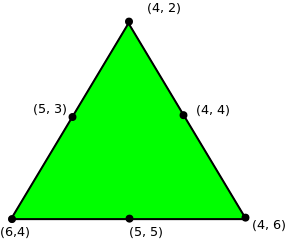Open in App
Not now

# Find coordinates of the triangle given midpoint of each side

• Last Updated : 27 Aug, 2022

Given three coordinates (x, y), which are the midpoint of the sides of the triangle. The task is to find the coordinates of the triangle.

Examples:

```Input : midx1 = 5, midy1 = 3
midx2 = 4, midy2 = 4
midx3 = 5, midy3 = 5

Output : x1 = 4 y1 = 2
x2 = 4 y2 = 6
x3 = 6 y3 = 4````Solution can be verified by the figure.`

Let’s separately solve for X-coordinates and Y-coordinates. For X coordinate of vertices, let them be x1, x2, x3. Then, X-coordinate of middle points will be (x1 + x2)/2, (x2 + x3)/2, (x3 + x1)/2. Observe, sum of these 3 expressions is equal to sum of X-coordinates. Now, we have sum of 3 variables and 3 expressions for sum of every pair of them, find out the values of coordinates by solving equations.
Similarly, we solve for Y-coordinates.

Below is the implementation of this approach:

## C++

 `// C++ program to find coordinate of the``// triangle given midpoint of each side``#include``#define N 3``using` `namespace` `std;` `// Return after solving the equations and``// finding the vertices coordinate.``vector<``int``> solve(``int` `v[])``{``    ``vector<``int``> res;` `    ``// Finding sum of all three coordinate.``    ``int` `all3 = v + v + v;` `    ``// Solving the equation.``    ``res.push_back(all3 - v*2);``    ``res.push_back(all3 - v*2);``    ``res.push_back(all3 - v*2);` `    ``return` `res;``}` `// Finds vertices of a triangles from given``// middle vertices.``void` `findVertex(``int` `xmid[], ``int` `ymid[])``{``    ``// Find X coordinates of vertices.``    ``vector<``int``> V1 = solve(xmid);` `    ``// Find Y coordinates of vertices.``    ``vector<``int``> V2 = solve(ymid);` `    ``// Output the solution.``    ``for` `(``int` `i = 0; i < 3; i++)``        ``cout << V1[i] << ``" "``             ``<< V2[i] <

## Java

 `import` `java.util.Vector;` `// Java program to find coordinate of the``// triangle given midpoint of each side``class` `GFG {` `//static final int N = 3;``// Return after solving the equations and``// finding the vertices coordinate.``    ``static` `Vector solve(``int` `v[]) {``        ``Vector res = ``new` `Vector();` `        ``// Finding sum of all three coordinate.``        ``int` `all3 = v[``0``] + v[``1``] + v[``2``];` `        ``// Solving the equation.``        ``res.add(all3 - v[``1``] * ``2``);``        ``res.add(all3 - v[``2``] * ``2``);``        ``res.add(all3 - v[``0``] * ``2``);` `        ``return` `res;``    ``}` `// Finds vertices of a triangles from given``// middle vertices.``    ``static` `void` `findVertex(``int` `xmid[], ``int` `ymid[]) {``        ``// Find X coordinates of vertices.``        ``Vector V1 = solve(xmid);` `        ``// Find Y coordinates of vertices.``        ``Vector V2 = solve(ymid);` `        ``// Output the solution.``        ``for` `(``int` `i = ``0``; i < ``3``; i++) {``            ``System.out.println(V1.get(i) + ``" "` `+ V2.get(i));``        ``}` `    ``}` `// Driver code``    ``public` `static` `void` `main(String[] args) {``        ``int` `xmid[] = {``5``, ``4``, ``5``};``        ``int` `ymid[] = {``3``, ``4``, ``5``};``        ``findVertex(xmid, ymid);``    ``}``}``// This code is contributed by``// PrinciRaj1992`

## Python3

 `# Python3 program to find coordinate of the``# triangle given midpoint of each side` `N ``=` `3` `# Return after solving the equations and``# finding the vertices coordinate.``def` `solve(v):` `    ``res ``=` `[]` `    ``# Finding sum of all three coordinate.``    ``all3 ``=` `v[``0``] ``+` `v[``1``] ``+` `v[``2``]` `    ``# Solving the equation.``    ``res.append(all3 ``-` `v[``1``] ``*` `2``)``    ``res.append(all3 ``-` `v[``2``] ``*` `2``)``    ``res.append(all3 ``-` `v[``0``] ``*` `2``)``    ``return` `res` `# Finds vertices of a triangles from given``# middle vertices.``def` `findVertex(xmid, ymid):` `    ``# Find X coordinates of vertices.``    ``V1 ``=` `solve(xmid)` `    ``# Find Y coordinates of vertices.``    ``V2 ``=` `solve(ymid)` `    ``# Output the solution.``    ``for` `i ``in` `range``(``0``, ``3``):``        ``print``(V1[i], end``=``" "``)``        ``print``(V2[i])` `# Driver code``if` `__name__``=``=``'__main__'``:``    ``xmid ``=` `[``5``, ``4``, ``5``]``    ``ymid ``=` `[``3``, ``4``, ``5``]``    ``findVertex(xmid, ymid)` `# This code is contributed by``# Sanjit_Prasad`

## C#

 `// C# program to find coordinate of the``// triangle given midpoint of each side``using` `System;``using` `System.Collections;` `class` `GFG``{` `    ``//static final int N = 3;``    ``// Return after solving the equations and``    ``// finding the vertices coordinate.``    ``static` `ArrayList solve(``int` `[]v)``    ``{``        ``ArrayList res = ``new` `ArrayList();` `        ``// Finding sum of all three coordinate.``        ``int` `all3 = v + v + v;` `        ``// Solving the equation.``        ``res.Add(all3 - v * 2);``        ``res.Add(all3 - v * 2);``        ``res.Add(all3 - v * 2);` `        ``return` `res;``    ``}` `    ``// Finds vertices of a triangles from given``    ``// middle vertices.``    ``static` `void` `findVertex(``int` `[]xmid, ``int` `[]ymid)``    ``{``        ``// Find X coordinates of vertices.``        ``ArrayList V1 = solve(xmid);` `        ``// Find Y coordinates of vertices.``        ``ArrayList V2 = solve(ymid);` `        ``// Output the solution.``        ``for` `(``int` `i = 0; i < 3; i++)``        ``{``            ``Console.WriteLine(V1[i] + ``" "` `+ V2[i]);``        ``}` `    ``}` `    ``// Driver code``    ``public` `static` `void` `Main()``    ``{``        ``int` `[]xmid = {5, 4, 5};``        ``int` `[]ymid = {3, 4, 5};``        ``findVertex(xmid, ymid);``    ``}``}` `// This code is contributed by mits`

## PHP

 ``

## Javascript

 ``

Output:

```6 4
4 2
4 6```

Time Complexity: O(1)

Auxiliary Space: O(1) because it is using constant space

This article is contributed by Anuj Chauhan. If you like GeeksforGeeks and would like to contribute, you can also write an article using write.geeksforgeeks.org or mail your article to review-team@geeksforgeeks.org. See your article appearing on the GeeksforGeeks main page and help other Geeks.